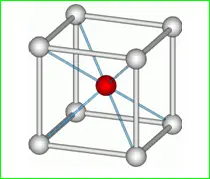# MCQs on Engineering Materials

##### Page 2 of 8. Go to page 1 2 3 4 5 6 7 8
01․ ALNICO is an alloy of
iron, aluminium.
aluminium, nickel.
iron, cobalt, nickel.
aluminium, nickel, iron and cobalt.

ALNICO is a exceptionally hard magnetic material, which is now a days used in making permanent magnets. It consists of Aluminium, nickel and cobalt (Al-ni-co).

02․ What is the name of the fluorescent material that gives red colour fluorescence?
Zinc silicate.
Calcium silicate.
Zinc sulphide.
Magnesium silicate.

The magnesium silicate material gives red colour fluorescence. The cerussite (lead carbonate) gives bright green colour fluorescence. The zinc arsenate gives bright colour fluorescence.

03․ Choose the best suitable of Diamagnetic : Paramagnetic::
Water : Air.
Iron : Steel.
Permalloy : Super-alloy.
Nickel : Cobalt.

Diamagnetic materials has negative susceptibility and paramagnetic substances has small positive susceptibility. Water is diamagnetic as it has weak attraction and air is paramagnetic as it has small attraction. Except Water and Air, all the above mentioned materials are the ferromagnetic type materials. Hence, the example material for diamagnetic is water and the example material for paramagnetic is air.

04․ Find the odd one out.
Absorptivity.
Emissivity.
Refractive index.
Dielectric strength.

Absorptivity, emissivity and refractive index the terms which are related with the optical systems. But, the dielectric strength is related with the capacitor (or) electrostatic field. Hence, the dielectric strength is the odd term of the above things.

05․ Which of the following is the least desired property in magnetic materials for electrical engineering applications ?
High magnetic permeability.
High electrical resistivity.
Large hysteresis loop.
All of the above.

High Permeability is desired because it helps in electromagnetic induction. High resistivity is required for good current conduction in conductor. But large hysteresis loop will increase hysteresis losses, so it is not desired. When a magnetic material is subjected to a cycle of magnetization (magnetized first in one direction and later demagnetized in opposite direction in a cyclic manner), energy loss takes place. This energy loss is due to molecular friction in the material. This energy loss is nothing but the hysteresis loss. So, for a large hysteresis loop, the point of hysteresis loss (or) magnetic saturation point is high. Hence, the effect of hysteresis loss is less in this case. That is why in the electrical applications, the magnetic material should not have larger value of hysteresis loop.

06․ Cryogenics is a branch of science dealing with which of the following?
High temperature.
Low temperature.
Friction and wear.
Crystal growth.

Cryogenics is the scientific study of the working of physical things at very low temperatures. Sometimes it approaches with zero degree temperature level and how such temperatures can be achieved and maintained is the main core of cryogenics.

07․ The numberof atoms per unit cell in a B.C.C structure is
7.
4.
2.
12.

We know that the cubic structure has totally 8 corners. Those 8 corners must have 8 atoms in each corner and there is one atom in the center of the cubic structure. The corner atoms are concentrated on the center atom. Hence, the total number of atoms in a BCC structure is 8. So, the number of atoms per unit cell in a BCC structure is [(1 / 8) × 8 ] + 1 = 2.08․ Substance having permeability less than the permeability of free space, are known as
ferromagnetic.
bipolar.
paramagnetic.
diamagnetic.

Diamagnetic material creates a magnetic field in opposition to an externally applied magnetic field and it is not a permanent magnet. Hence, its magnetic permeability is less than the permeability of free space.

09․ Susceptibility is negative for
non magnetic substances.
diamagnetic substances.
ferromagnetic substances.
none of above.

Magnetic susceptibility is the degree of magnetization of a material response to an applied magnetic field. If magnetic susceptibility is positive then the material can be paramagnetic, ferromagnetic or anti ferromagnetic. Alternatively, if the magnetic susceptibility is negative, then the material is diamagnetic.

10․ The bar magnet has
the dipole moment.
monopole moment.
(A) and (B) both.
none of above.

According to the modern theory, a bar magnet has infinite no of small dipole moment.

<<<1234>>>